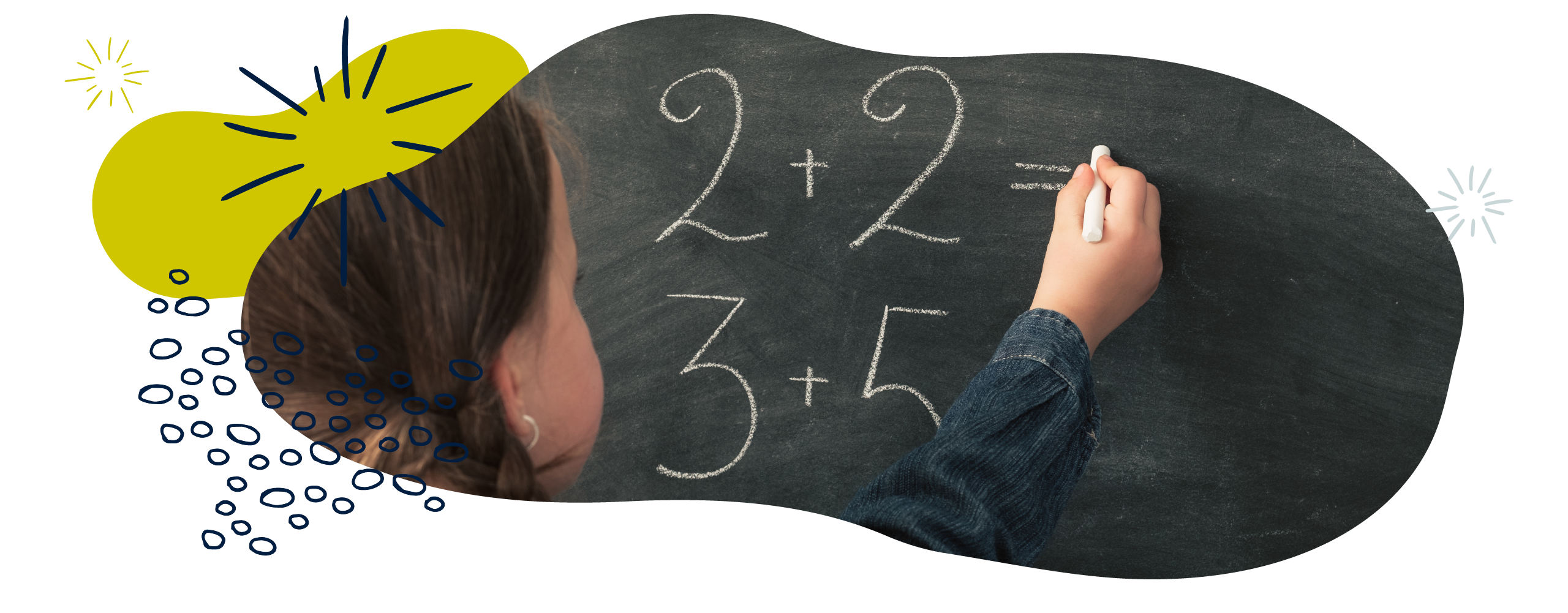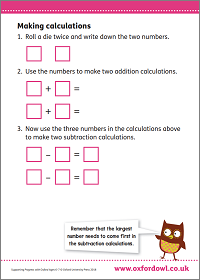We use cookies to enhance your experience on our website. By continuing to use our website, you are agreeing to our use of cookies. You can change your cookie settings at any time. Find out more# Addition & subtraction in Year 2 (age 6–7)

In Year 2, your child will solve addition and subtraction problems involving numbers, quantities, and measures. They will use a range of objects, pictures, diagrams, and symbols such as ‘+’, ‘–’ and ‘=’.

The key words for this section are commutative, difference, and place value.

## What your child will learn

Take a look at the National Curriculum expectations for addition and subtraction in Year 2 (ages 6–7):

#### Solve problems with addition and subtraction

Your child will use objects (like counters, straws, and fruit), pictures (like number lines, grids, and arrays), and symbols (‘+’, ‘–’, and ‘=’) to solve maths problems.

Your child will encounter problems that involve:

• Numbers
• Quantities (for example, ‘3 apples’)
• Measures (for example, ‘5 centimetres’).

Your child’s increasing knowledge of mental and written methods will give them lots of different ways to do this. They will choose a method that best fits any given problem.

#### Use addition and subtraction facts up to 20, and derive and use related facts up to 100

Your child will know and use addition and subtraction facts up to 20. Then, they will use their knowledge of number facts and their knowledge of place value to help them work out addition and subtraction facts up to 100. For example:

If children know that 2 + 6 = 8, then they can work out that 20 + 60 = 80.

2 has become ten times larger and 6 has become ten times larger, so the total will be ten times larger.

In the above example, try to avoid telling your child that they ‘add a zero’, as this does not help them with their understanding of number and place value. It might end up being more confusing when children are also learning that adding zero does not change a number, so, for example, 2 + 0 = 2.

Your child will be taught to look for all sorts of patterns in numbers to help them solve problems. For example:

If your child knows 3 + 4 = 7, can they use this knowledge to work out the answer to 13 + 4 = ?

Your child should notice that one ten has been added to the 3, so the total will increase by one ten.

#### Add and subtract numbers mentally, and using objects and pictures

Your child will add and take away lots of combinations of numbers, including:

• a two-digit number and 1s (for example, 42 +/– 4)
• a two-digit number and 10s (for example, 34 +/– 20)
• 2 two-digit numbers (for example, 56 +/– 37)
• adding 3 one-digit numbers (for example, 3 + 9 + 5).

Your child will learn to add and subtract numbers using objects, pictures, and symbols, and also to solve simple problems mentally. They will be expected to explain how they have solved problems.

#### Show that addition can be done in any order and subtraction cannot

Your child will learn that when they are adding numbers, they get the same total no matter what order they add the numbers together:

7 + 4 = 11

4 + 7 = 11

This means that addition is commutative.

With subtraction, the order of the numbers does matter, as we will get different answers:

7 – 4 = 3

4 – 7 = –3

This means that subtraction is not commutative.

#### Use the inverse relationship between addition and subtraction to solve and check calculations

Your child will be taught to understand the inverse relationship between addition and subtraction, i.e. that addition and subtraction undo each other.

They will be expected to use their understanding of this inverse relationship to check their calculations. For example, if they have calculated 5 + 6 = 11, they can check their answer by solving 11 – 6 = 5 or 11 – 5 = 6.

## How to help at home

There are lots of quick and easy ways that you can help your child with addition and subtraction. Here are just a few everyday ideas to support your child’s learning:

### 1. Use the language of addition and subtraction

Using the correct mathematical vocabulary will get your child used to deconstructing word problems, and will make them familiar with the kinds of words they will hear in school.

Try to use mathematical language like add, altogether, more, plus, total, and sum when adding. When your child finds the answer to an addition calculation, encourage them to use the word total or sum. If they talk about the fact that you can add numbers in any order and get the same answer, encourage them to use the word commutative.

There is also a lot of mathematical vocabulary related to subtraction. When your child tells you the answer to a subtraction calculation, encourage them to refer to this as the difference. For example, ‘7 – 3 = 4’ could be read as ‘the difference between 7 and 3 is 4’. They should also try to use the language take away, subtract, minus, less, fewer, and so on.

Try writing out the addition and subtraction symbols (‘+’ and ‘–’) along with the related words on cards (for example, add, altogether, more, plus, total, sum, take away, subtract, minus, less, and fewer). Help your child to link the words to the correct symbol. If necessary, help them to read some of the words.

### 2. Play the Addition and Subtraction Facts game

Encourage your child to practise addition and subtraction to 20. This will help them derive related facts such as:

3 + 7 = 10

10 – 7 = 3

7 = 10 – 3.

Once they’ve done this, they should be able to use their knowledge of place value to calculate more facts:

30 + 70 = 100

100 – 70 = 30

70 = 100 – 30.

Try giving your child a simple calculation such as 5 + 3 = 8. Ask them to record as many related addition and subtraction facts as they know. For example, if they know that 5 + 3 = 8, then they should be able to work out that 3 + 5 = 8,  8 – 3 = 5, 8 – 5 = 3, 15 + 3 = 18, 50 + 30 = 80, and so on.

### Activity: Making calculationsUse dice to make your own calculations.

### 3. Try lots of different methods

Encourage your child to use a range of methods, including using objects, drawings, diagrams, and symbols, to solve addition and subtraction problems. To solve addition problems, your child might:

• Use objects. They could count all the objects in groups being added together to find their total (so for 2 + 3, they would count all the objects together: 1, 2, 3, 4, 5). Then, then could begin counting on from one group to find the total (so for 2 + 3, they might start at 3 and count on: 3, 4, 5).
• Use drawings such as number lines.
• Use formal written methods such as column addition.

To solve subtraction problems, your child might:

• Use objects and take some away to find the difference.
• Put objects from two groups into two lines and compare them to find the difference.
• Count backwards or use number lines.
• Use written methods such as column subtraction.

Your child could use rounding to estimate their answers before calculating accurately. It can also be helpful to use more than one method to double-check calculations. For example, your child could check a subtraction question by adding up the answer and the number they subtracted to see if they get back to the number they started from.

#### Choose an addition or subtraction calculation to solve with your child

We are setting the table for our family of four and one guest. How many people are eating altogether?

I’ve put out 8 forks. Is that right or is it too many? How many do we need to put back in the drawer?

You have sent 20 invitations out for your party. We have heard back from everybody and 3 people have told us they can’t come. How many people are coming to your party?

Discuss which method your child would use for these questions. Try to find as many ways as you can to solve the problems, and talk about the methods you and your child each preferred and why.

### 4. Make up story problems

Story problems can be a fun way to help your child understand addition and subtraction. And you can have fun making them up! Why not see if your child would like to create a story problem for you?

When creating an addition story problem, you could support your child by giving them objects to use or a picture to inspire their story. Once they’ve completed the word problem, ask them if they could write the calculations in the problem using symbols (‘+’, ‘–’, and ‘=’).

### 5. Try missing number problems

Missing number problems can be quite tricky for children. You can support your child by making the most of opportunities to practise missing number problems practically. For example, you can ask for your child’s help if you have a number of something but need a higher number:

‘I have 5 buttons but I need 15 buttons. How many more do I need?’

Ask your child how they worked it out. Then, you could ask them to write a calculation using numbers and symbols to explain how they did it.

5 + ? = 15.

They could have counted on from 5 to 15 to get 10.

They could have counted back 5 from 15 to get 10.

They may have known that 15 – 5 = 10.

… and so on.